# Fast.ai Deep Learning Part 1— Lesson 6 My Personal Notes.“turned on gray and white Google Home Mini speaker on white surface” by Kevin Bhagat on Unsplash

Summary: After this course you should understand how Recurent Neural Network (RNN) are working. It is important part of natural language progressing.

Code

Video

First let’s start with cool Python trick. Write `@property` top of a method and you can call it without parenthesis.

`@propertydef test():    return "Hello"print(test)OUTPUT: Hello`

To plot our data we often need to reduce dimensionality. One of the most common technique is PCA.

`from sklearn.decomposition import PCApca = PCA(n_components=3)data_pca = pca.fit(data).components_`

PCA reduce the number of dimensions by combining the most similar dimensions. Result dimensions are as different as plausible. It is not very important to understand this because scikit-learn is doing it for us.

dense layer = linear layer

Jeremy showed from some paper how using embedding matrix (EE) will help all different kind of models. He explained that if you make your data to use embedding matrix it can be easily given anyone with different kind of models and they all got pretty good results. In this sheet lower is better.

Don’t touch any column just because it looks like irrelevant! Always first analyse it and after that you might delete it.

Deleting columns doesn’t help a lot in deep learning so it is better to just give the model to choose which to delete and which to leave.

[1:10:07]

Recurrent Neural Network (RNN)

RNN is like normal neural network but it is built a way that it can remember old things. Like if in sentence there is “a man” at the beginning then RNN can later on realize that it is “he” not “she” or “it”.

Code of this chart:

First we input the data

`PATH = 'data/nietzsche/'get_data("https://s3.amazonaws.com/text-dataset/nietzsche.txt",f'{PATH}'nietzsche.txt)text = open(f'{PATH}nietzsche.txt').read()print('corpus length:',len(text))OUTPUT: 600893chars = sorted(list(set(text)))vocab_size = len(chars)+1print('total chars:',vocab_size)OUTPUT: 85`

Map every character to unique id (1. row) and every unique id to character (2. row).

`char_indices = dict((c, i) for i, c in enumerate(chars))indices_char = dict((i, c) for i, c in enumerate(chars))`

Next we will convert all character in the text to their index

`idx = [char_indices[c] for c in text]idx[:5]OUTPUT: [40, 42, 29, 30, 25]`

We try to predict 4th character using 3 earlier characters.

`cs = 8c_in_dat = [[idx[i+j] for i in range(cs)] for j in range(len(idx)-                         cs-1)]c_out_dat = [idx[j+cs] for j in range(len(idx)-cs-1)]`

Inputs

`xs = np.stack(c_in_dat,axis=0)`

Outputs

`y = np.stack(c_out_dat)`

Dimensions

`x.shapeOUTPUT: (600884,8)`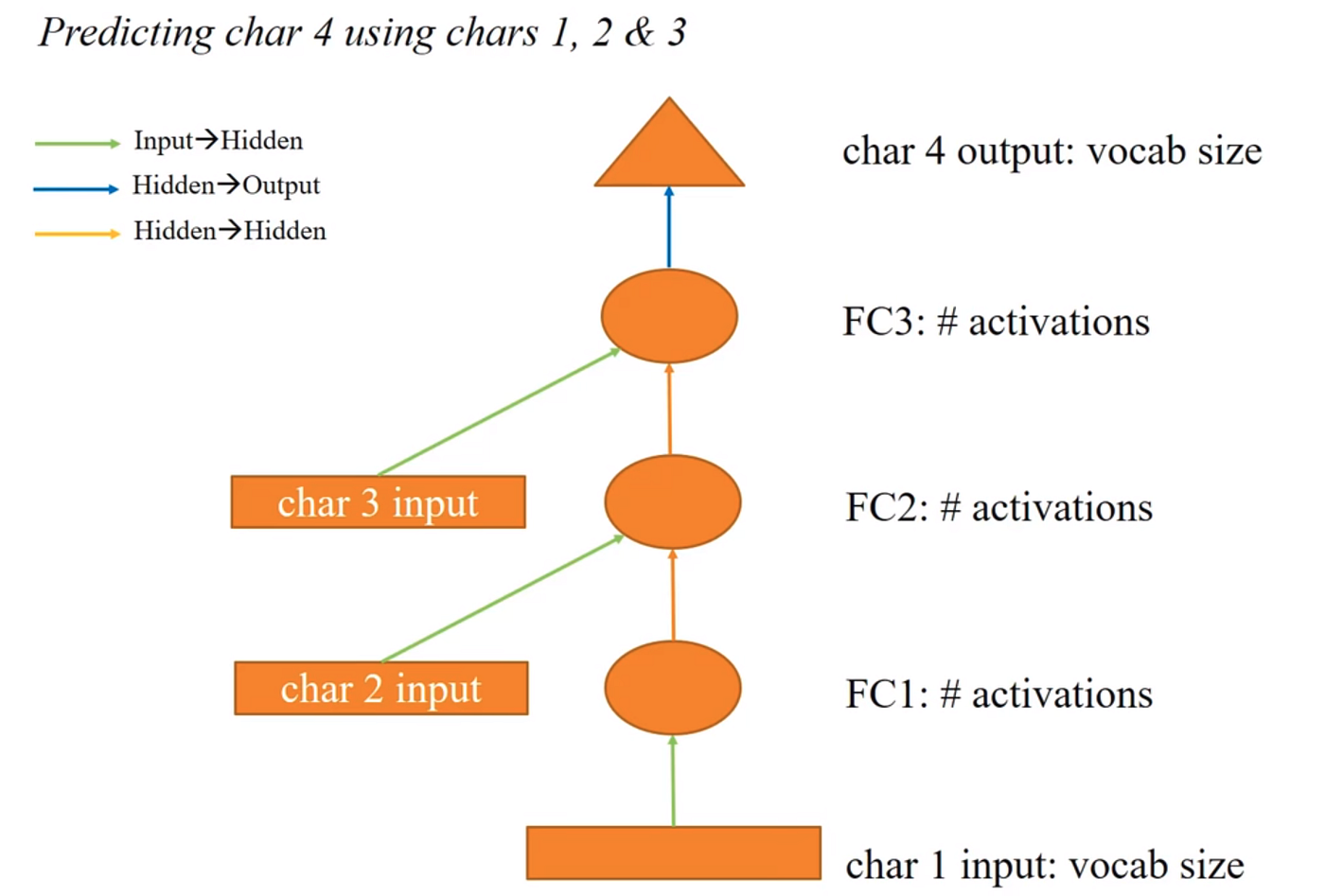All inputs are using the same weight matrix. We have 3 different weight matrix in this model.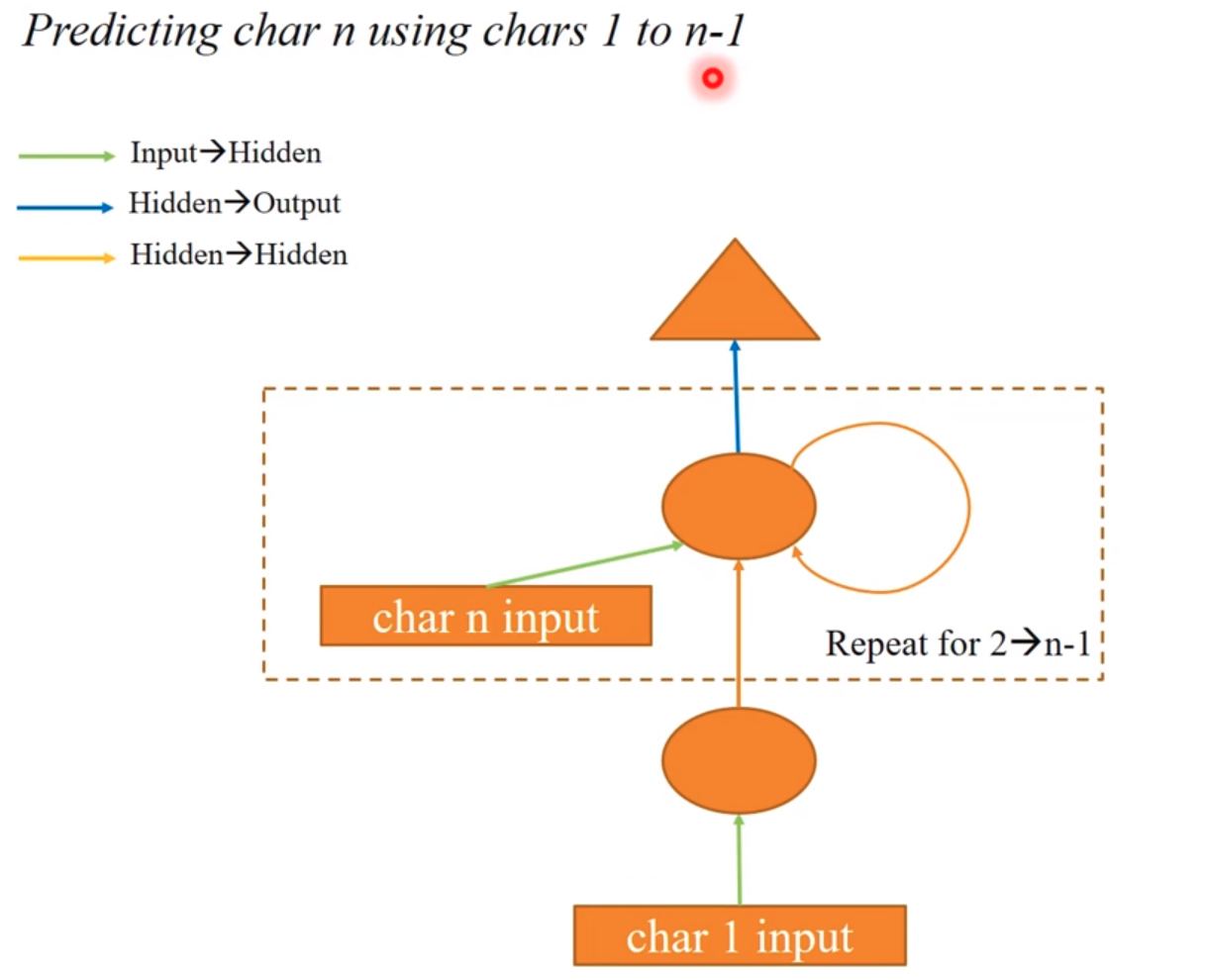Same thing but now we made the model more flexible.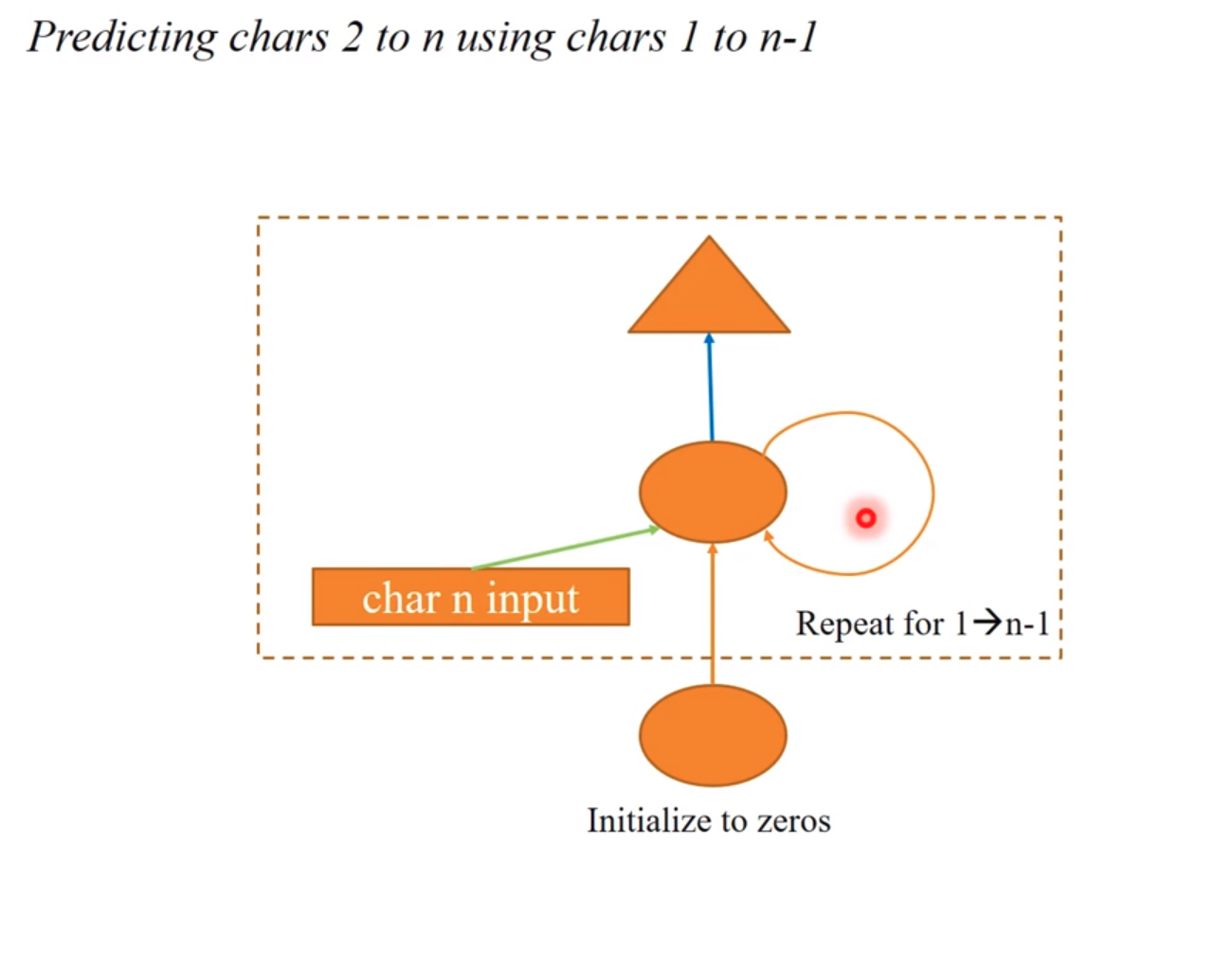We continue simplifying the model. Remember this is the same model than two above.

Next we will create that model above and train it.

`n_hidden = 256n_fac = 42val_idx = get_cv_idxs(len(idx)-cs-1)md = ColumnarModelData.from_arrays('.',val_idx,xs,y,bs=512)class Char3Model(nn.Module):    def __init__(self,vocab_size,n_fac):        super().__init__()        self.e = nn.Embedding(vocab_size,n_fac)                self.l_in = nn.Linear(n_fac+n_hidden, n_hidden)        self.l_hidden = nn.Linear(n_hidden, n_hidden)        self.l_out = nn.Linear(n_hidden, vocab_size)    def forward(self,c1,c2,c3):        bs = cs.size(0)        h = V(torch.zeros(bs,n_hidden).cuda())        for c in cs:            inp = torch.cat((h,self.e(c)),1)            inp = F.relu(self.l_in(inp))            h = F.tanh(self.l_hidden(inp))        return F.log_softmax(self.l_out(h))m = Char3Model(vocab_size,n_fac).cuda()it = iter(md.trn_dl)*xs,yt = nex(it)t = m(*V(xs))opt = optim.Adam(m.parameters(),1e-2)fit(m,md,1,opt,F.nll_loss)set_lrs(opt,0.001)fit(m,md,1,opt,F.nll_loss)`

Next we will write little code which we can use to test this model

`def get_next(inp):    idxs = T(np.array([char_indices[c] for c in inp]))    p = m(*VV(idxs))    i = np.argmax(to_np(p))    return chars[i]get_next('y. ')OUTPUT: 'T'get_next('and')OUTPUT: ' 'get_next('part of')OUTPUT: 't'`

[1:48:50]

Exact same thing using PyTorch

`class CharRNN(nn.Module):    def __init__(self,vocab_size,n_fac):        super().__init__()        self.e = nn.Embedding(vocab_size,n_fac)        self.rnn = nn.RNN(n_fac,n_hidden)        self.l_out = nn.Linear(n_hidden, vocab_size)    def forward(self, *cs):        bs = cs.size(0)        h = V(torch.zeros(1,bs,n_hidden))        inp = self.e(torch.stack(cs))        output,h = self.rnn(inp,h)        return F.log_softmax(self.l_out(outp[-1]))m = CharRnn(vocab_size,n_fac).cuda()opt = optim.Adam(m.parameters(),1e-3)it = iter(md.trn_dl)*xs,yt = next(it)t = m.e(V(torch.stack(xs)))ht = V(torch.zeros(1,512,n_hidden))outp, hn = m.rnn(t,ht)t = m(*V(xs))fit(m,md,1,opt,F.nll_loss)`

Using identity matrix as default numbers to hidden layers is great way to improve the model. In PyTorch this line do it and results are much better.

`m.rnn.weight_hh_10.data.copy_(torch.eye(n_hidden))`

~Lankinen

--

--

--

## More from Lankinen

Love podcasts or audiobooks? Learn on the go with our new app.

## Artificial Neural Network (NN) Explained in 5 Minutes with Animations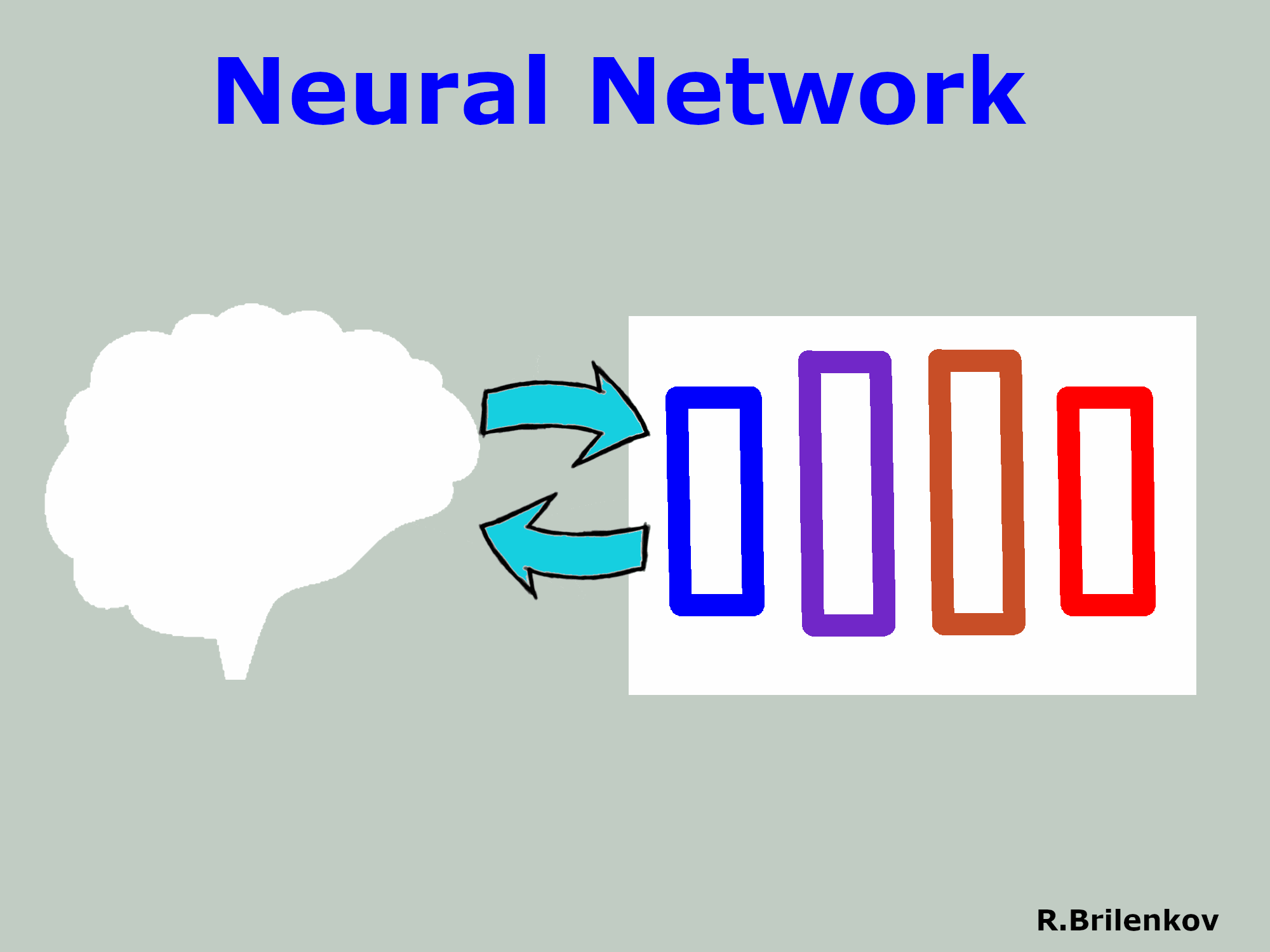## Beyond Weisfeiler-Lehman## Using CNNS to Detect Malaria in Blood Cells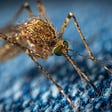## What Is Transfer Learning## Biologists and Data Scientists: The Cultural Divide## Covid-19 Detection by analyzing Chest X-Ray Images-I## Is this the real life? Is this just Generative Adversarial Network?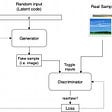## Unsupervised Learning: Explained, Briefly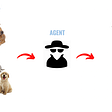## Machine Learning in Medicine — Journal Club## Breast Cancer classification using machine learning